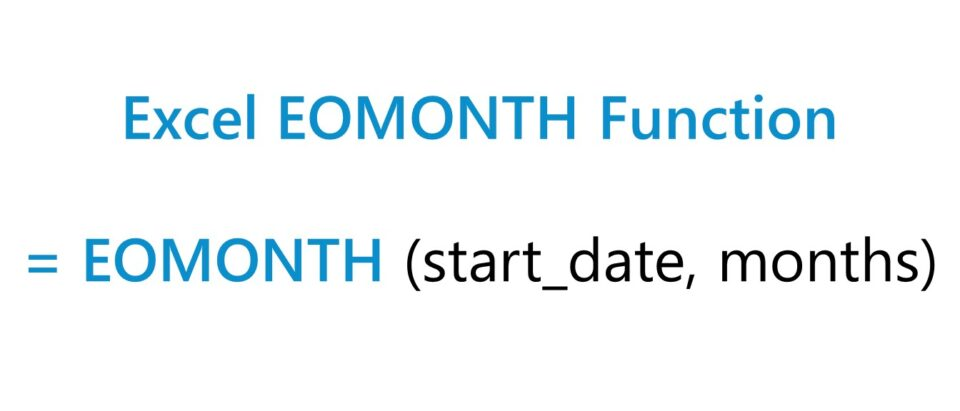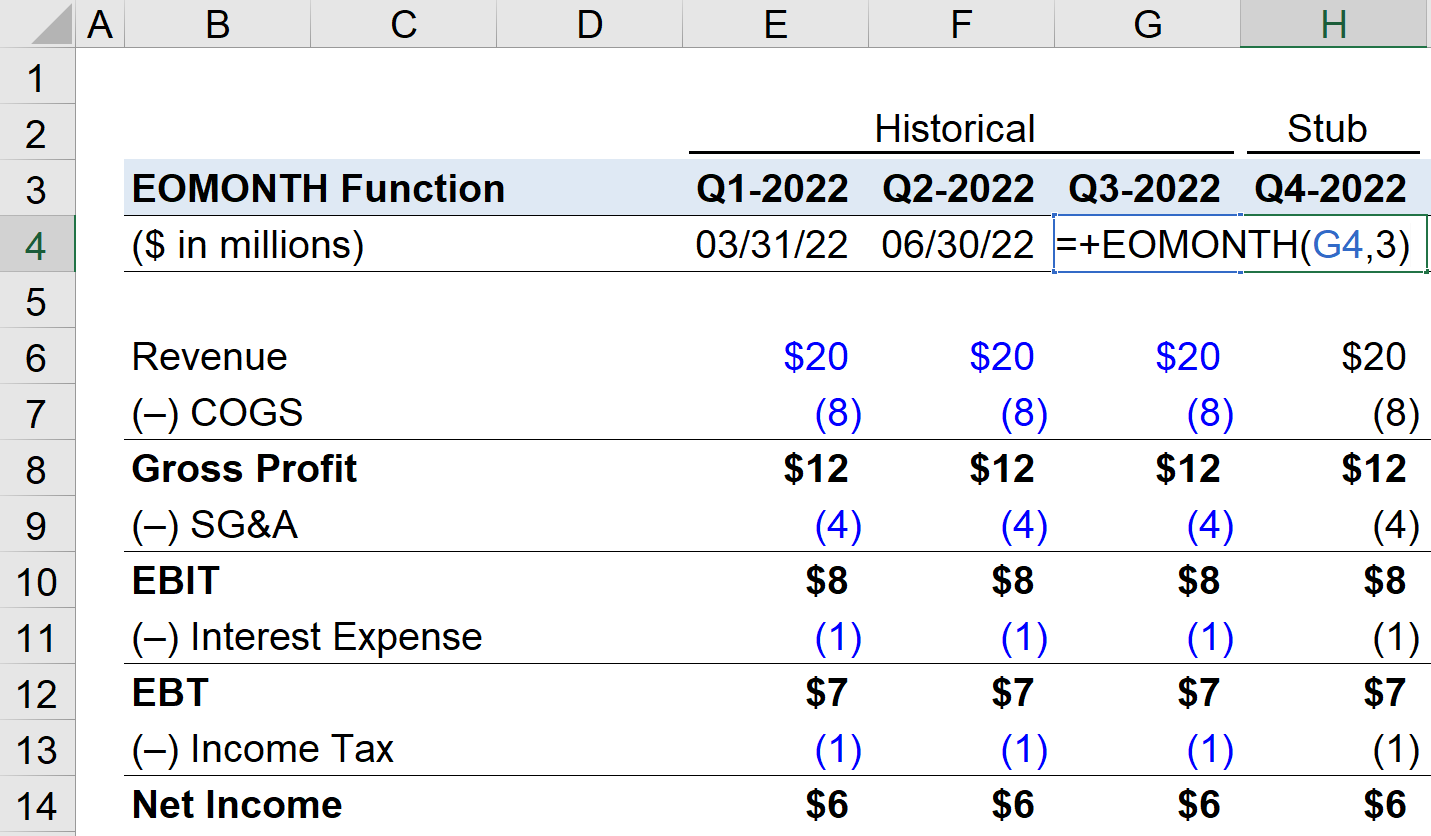Welcome to Wall Street Prep! Use code at checkout for 15% off.# EOMONTH Function

Guide to Understanding the “EOMONTH” Function in Excel## How to Use EOMONTH Function in Excel (Step-by-Step)

The Excel EOMONTH function stands for “End of Month” and is used to determine the end date given a specified time interval.

The EOMONTH function is a built-in feature of Excel frequently used in financial modeling because it conveniently automates the process of finding a specific date in either the past or the future.

For example, a quarterly projection model can utilize the function to quickly calculate the calendar date that falls on the last day of the next quarter, i.e. three months from the current month.

## Excel EOMONTH Function Formula

The Excel EOMONTH function formula is as follows.

=EOMONTH(start_date, months)
• start-date” → The initial date from which the monthly interval starts.
• months” → The number of months before or after the starting date.

## EOMONTH Function Syntax

The “months” argument specifies the number of months into the future or the past to calculate.

• Positive Integer → If a positive integer is entered into the “months” argument, the returned date will be on a forward basis, i.e. into the future, so entering “12” would calculate the final date on the month twelve months later from the starting date.
• Negative Integer → In contrast, a negative integer yields the date on a backward basis, so entering “-12” would result in the final date of the month twelve months ago.

Note that an error message appears if the starting date is not an actual date on the calendar. For example, a hard-coded date entry of February 29 on a non-leap year would not work.

To reduce the potential for any error messages, it is recommended for the starting date to be an automatic calculation and cell reference, rather than a manual entry.

The returned date values automatically adjust based on the calendar of each year, making the function far more reliable than manual entries.

The ending date is also, at all times, the last day of the month, irrespective of whether the starting date is the final date of the month or not (e.g. 11/15/2022).

## Months Argument: Monthly, Quarterly, and Annual Model

The following table describes the “months” argument corresponding to the periodicity of the most common types of financial models, assuming forward-looking projections (i.e. positive integers).

Periodicity Months Description
Monthly Model
• “12”
• The final date of the month, after one month has passed.
Quarterly Model
• “3”
• The final date of the month, once three months have passed.
Semi-Annual Model
• “2”
• The final date of the month, after six months have passed.
Annual Model
• “1”
• The final date of the month, after twelve months have passed, i.e. one fiscal year.

## EOMONTH Function Calculator – Excel Template

We’ll now move on to a modeling exercise, which you can access by filling out the form below.Submitting...

## EOMONTH Function Calculation Example

Suppose we’re forecasting the financials of a public company to create a five-year annual model.

The date of the company’s latest quarterly report was in Q-3 of 2022, which was September 30, 2022.

The first step in preparing our 5-year annual financial model is to project the remaining quarter in the current year (i.e. Q-4 of 2022) before shifting to a fiscal year basis.

The arguments in our formula for the Q-4 2022 date cell – the stub period – are the following:

=EOMONTH(G4,3)The returned date is 12/30/2022, the final date marking the end of fiscal year 2022.

In the column to the right, we’ll calculate the sum of the quarterly financials to arrive at our FY-2022 projection (and the cell containing the date will reference the cell from Q-4 2022).

From that point onward, we’ll adjust our monthly input to “12” and drag the formula across for the next four years (and have five projected years in total).

=EOMONTH(I4,12)

Turbo-charge your time in Excel Used at top investment banks, Wall Street Prep's Excel Crash Course will turn you into an advanced Power User and set you apart from your peers.